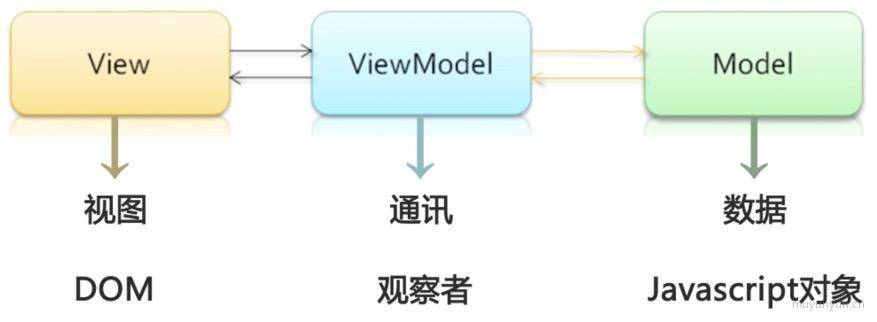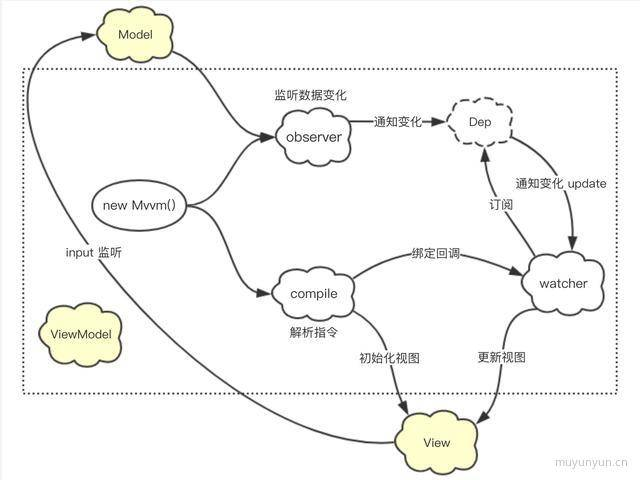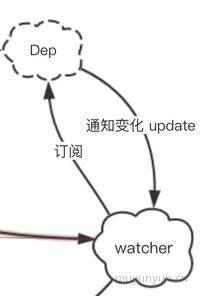# 前端MVVM框架解析之双向绑定

MVVM框架近年来前端一个明显的开发趋势就是架构从传统的MVC模式向MVVM模式迁移。在传统的M

MVVM 框架

MVVM 框架基本概念MVVM 框架的应用场景

MVVM 框架的好处显而易见：当前端对数据进行操作的时候，可以通过 Ajax 请求对数据持久化，只需改变 dom 里需要改变的那部分数据内容，而不必刷新整个页面。特别是在移动端，刷新页面的代价太昂贵。虽然有些资源会被缓存，但是页面的 dom、css、js 都会被浏览器重新解析一遍，因此移动端页面通常会被做成 SPA 单页应用。由此在这基础上诞生了很多 MVVM 框架，比如 React.js、Vue.js、Angular.js 等等。

MVVM 框架的简单实现Mvvm(入口文件) 的实现

``````
const vm = new Mvvm({
el: '#app',
data: {
title: 'mvvm title',
name: 'mvvm name'
},
})``````

``````
function Mvvm (options) {
this.data = options.data
const self = this
Object.keys(this.data).forEach(key =>
self.proxyKeys(key)
)
}
Mvvm.prototype = {
proxyKeys: function(key) {
const self = this
Object.defineProperty(this, key, {
get: function () { // 这里的 get 和 set 实现了 vm.data.title 和 vm.title 的值同步
return self.data[key]
},
set: function (newValue) {
self.data[key] = newValue
}
})
}
}
``````

``````
function Mvvm (options) {
this.data = options.data
// ...
observe(this.data)
new Compile(options.el, this)
}
``````

observer(观察者) 的实现

observer 的职责是监听 Model(JS 对象) 的变化，最核心的部分就是用到了 Object.defineProperty() 的 get 和 set 方法，当要获取 Model(JS 对象) 的值时，会自动调用 get 方法；当改动了 Model(JS 对象) 的值时，会自动调用 set 方法；从而实现了对数据的劫持，代码如下所示。

``````
let data = {
number: 0
}
observe(data)
data.number = 1 // 值发生变化
function observe(data) {
if (!data || typeof(data) !== 'object') {
return
}
const self = this
Object.keys(data).forEach(key =>
self.defineReactive(data, key, data[key])
)
}
function defineReactive(data, key, value) {
observe(value) // 遍历嵌套对象
Object.defineProperty(data, key, {
get: function() {
return value
},
set: function(newValue) {
if (value !== newValue) {
console.log('值发生变化', 'newValue:' + newValue + ' ' + 'oldValue:' + value)
value = newValue
}
}
})
}
``````

Dep(订阅者数组) 和 watcher(订阅者) 的关系``````
// observer.js
function Dep() {
this.subs = [] // 存放订阅者
}
Dep.prototype = {
this.subs.push(sub)
},
notify: function() { // 通知订阅者更新
this.subs.forEach(function(sub) {
sub.update()
})
}
}
function observe(data) {...}
function defineReactive(data, key, value) {
var dep = new Dep()
observe(value) // 遍历嵌套对象
Object.defineProperty(data, key, {
get: function() {
if (Dep.target) { // 往订阅器添加订阅者
}
return value
},
set: function(newValue) {
if (value !== newValue) {
console.log('值发生变化', 'newValue:' + newValue + ' ' + 'oldValue:' + value)
value = newValue
dep.notify()
}
}
})
}
``````

``````
// watcher.js
function Watcher(vm, exp, cb) {
this.vm = vm
this.exp = exp
this.cb = cb
this.value = this.get()
}
Watcher.prototype = {
update: function() {
this.run()
},
run: function() {
// ...
if (value !== oldVal) {
this.cb.call(this.vm, value) // 触发 compile 中的回调
}
},
get: function() {
Dep.target = this // 缓存自己
const value = this.vm.data[this.exp] // 强制执行监听器里的 get 函数
Dep.target = null // 释放自己
return value
}
}
``````

1 在自身实例化的过程中，往订阅器(dep) 中添加自己

2 当 model 发生变动，dep.notify() 通知时，其能调用自身的 update 函数，并触发 compile 绑定的回调函数实现视图更新

compile(编译) 的实现

``````
function Compile(el, vm) {
this.vm = vm
this.el = document.querySelector(el)
this.fragment = null
this.init()
}
Compile.prototype = {
init: function() {
if (this.el) {
this.fragment = this.nodeToFragment(this.el) // 将节点转为 fragment 文档碎片
this.compileElement(this.fragment) // 对 fragment 进行编译解析
this.el.appendChild(this.fragment)
}
},
nodeToFragment: function(el) {
const fragment = document.createDocumentFragment()
let child = el.firstChild // △ 第一个 firstChild 是 text
while(child) {
fragment.appendChild(child)
child = el.firstChild
}
return fragment
},
compileElement: function(el) {...},
}
``````

``````
Compile.prototype = {
init: function() {
if (this.el) {
this.fragment = this.nodeToFragment(this.el) // 将节点转为 fragment 文档碎片
this.compileElement(this.fragment) // 对 fragment 进行编译解析
this.el.appendChild(this.fragment)
}
},
nodeToFragment: function(el) {...},
compileElement: function(el) {...},
compileText: function (node, exp) { // 对文本类型进行处理，将 {{abc}} 替换掉
const self = this
const initText = this.vm[exp]
this.updateText(node, initText) // 初始化
new Watcher(this.vm, exp, function(value) { // 实例化订阅者
self.updateText(node, value)
})
},
compileEvent: function (node, vm, exp, dir) { // 对事件指令进行处理
const eventType = dir.split(':')
const cb = vm.methods && vm.methods[exp]
if (eventType && cb) {
}
},
compileModel: function (node, vm, exp) { // 对 v-model 进行处理
let val = vm[exp]
const self = this
this.modelUpdater(node, val)
const newValue = e.target.value
self.vm[exp] = newValue // 实现 view 到 model 的绑定
})
},
}
``````